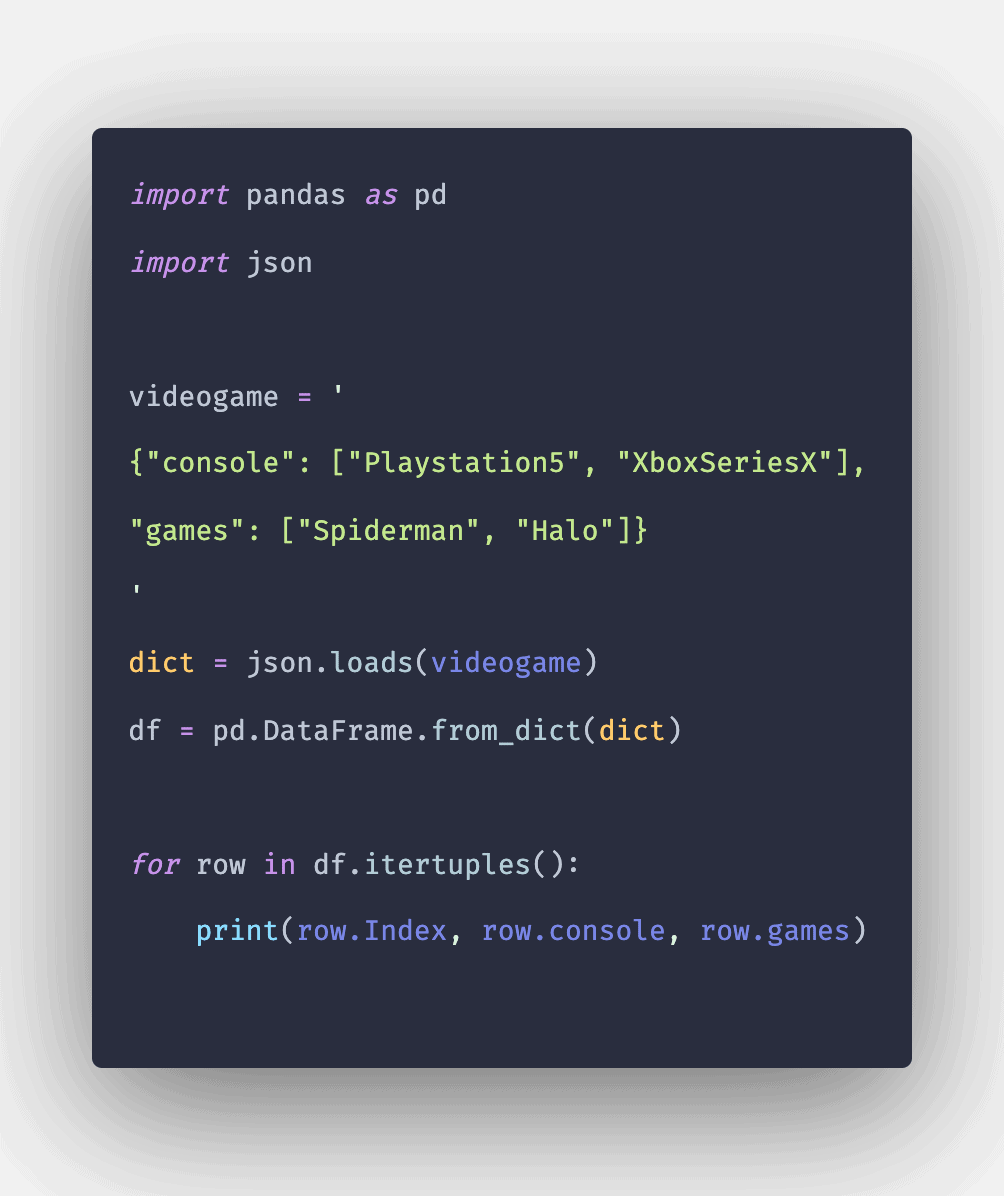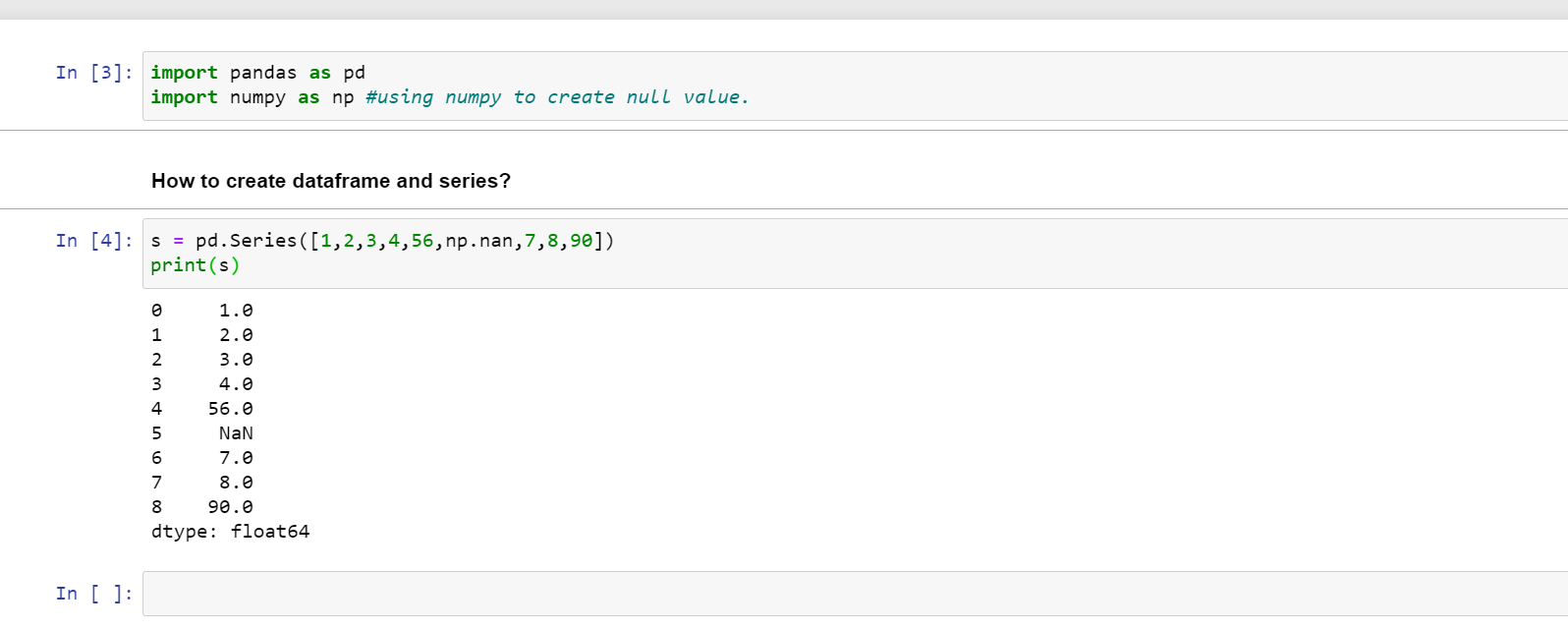1590439380

# Pandas Iterrows: How To Iterate Over Pandas Rows

Pandas iterrows is an inbuilt DataFrame function that will help you loop through each row. Pandas iterrows() method returns an iterator containing the index of each row and the data in each row as a Series. Since iterrows() returns an iterator, we can use the next function to see the content of the iterator.

#python #pandas

## Buddha Community1586702221

## Python Pandas Objects - Pandas Series and Pandas Dataframe

In this post, we will learn about pandas’ data structures/objects. Pandas provide two type of data structures:-

### Pandas Series

Pandas Series is a one dimensional indexed data, which can hold datatypes like integer, string, boolean, float, python object etc. A Pandas Series can hold only one data type at a time. The axis label of the data is called the index of the series. The labels need not to be unique but must be a hashable type. The index of the series can be integer, string and even time-series data. In general, Pandas Series is nothing but a column of an excel sheet with row index being the index of the series.

### Pandas Dataframe

Pandas dataframe is a primary data structure of pandas. Pandas dataframe is a two-dimensional size mutable array with both flexible row indices and flexible column names. In general, it is just like an excel sheet or SQL table. It can also be seen as a python’s dict-like container for series objects.

#python #python-pandas #pandas-dataframe #pandas-series #pandas-tutorial1602550800

## Pandas in Python

Pandas is used for data manipulation, analysis and cleaning.

What are Data Frames and Series?

Dataframe is a two dimensional, size mutable, potentially heterogeneous tabular data.

It contains rows and columns, arithmetic operations can be applied on both rows and columns.

Series is a one dimensional label array capable of holding data of any type. It can be integer, float, string, python objects etc. Panda series is nothing but a column in an excel sheet.

### How to create dataframe and series?

s = pd.Series([1,2,3,4,56,np.nan,7,8,90])

print(s)How to create a dataframe by passing a numpy array?

1. d= pd.date_range(‘20200809’,periods=15)
2. print(d)
3. df = pd.DataFrame(np.random.randn(15,4), index= d, columns = [‘A’,’B’,’C’,’D’])
4. print(df)

#pandas-series #pandas #pandas-in-python #pandas-dataframe #python1616050935

## WORKING WITH GROUPBY IN PANDAS

In my last post, I mentioned the groupby technique  in Pandas library. After creating a groupby object, it is limited to make calculations on grouped data using groupby’s own functions. For example, in the last lesson, we were able to use a few functions such as mean or sum on the object we created with groupby. But with the aggregate () method, we can use both the functions we have written and the methods used with groupby. I will show how to work with groupby in this post.

#pandas-groupby #python-pandas #pandas #data-preprocessing #pandas-tutorial1589781660

## Pandas Iterrows: How To Iterate Over Pandas Rows

Pandas iterrows is an inbuilt DataFrame function that will help you loop through each row. Pandas iterrows() method returns an iterator containing the index of each row and the data in each row as a Series. Since iterrows() returns an iterator, we can use the next function to see the content of the iterator.

Pandas Iterrows

Pandas iterrows() function is used to to iterate over rows of the Pandas Dataframe. In addition to iterrows, Pandas also has a useful function itertuples(). The iterrows() function is used to iterate over DataFrame rows as (index, Series) pairs. The function Iterates over the DataFrame columns, returning the tuple with the column name and the content as a Series.

#pandas

1590439380

## Pandas Iterrows: How To Iterate Over Pandas Rows

Pandas iterrows is an inbuilt DataFrame function that will help you loop through each row. Pandas iterrows() method returns an iterator containing the index of each row and the data in each row as a Series. Since iterrows() returns an iterator, we can use the next function to see the content of the iterator.

#python #pandas